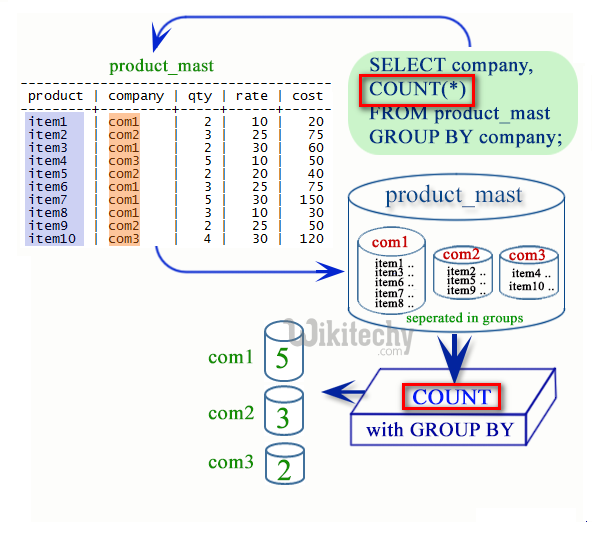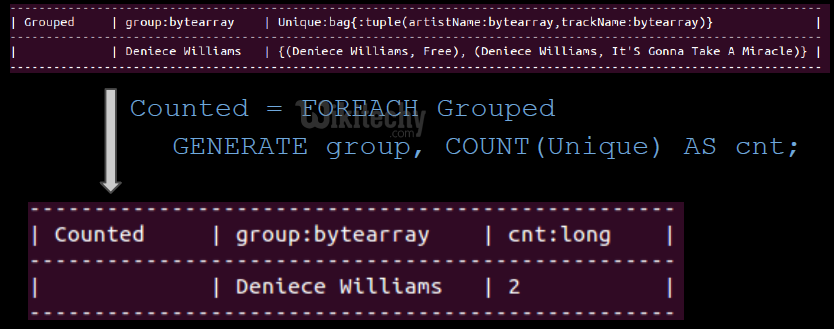# pig tutorial - apache pig tutorial - Apache Pig COUNT() Function - pig latin - apache pig - pig hadoop

## What is Count Function in Apache Pig ?

• The COUNT() function used in Apache Pig is used to get the number of elements in a bag.
• The COUNT() function ignores all the tuples which is having a NULL value in the first field while counting the number of tuples given in a bag
• The COUNT() function returns the number of rows that matches a specified criteria.
• The COUNT() function returns the number of values which is given in a set of values.
• The COUNT() function counts the number of cells that contain numbers, and also counts the numbers which are written within the list of arguments.Learn Apache Pig - Apache Pig tutorial - Apache Pig Count() Function - Apache Pig examples - Apache Pig programs

## Pig Operations - Aggregation

• build-in functions e.g. AVG, COUNT, COUNT_STAR, MAX, MIN, SUM
• possibility to implement custom UDFs
•## Example

wikitechy_employee_details.txt

• We have loaded the into Pig with the relation name wikitechy_employee_details which is given below:

## Calculating the Number of Tuples

• We can use the built-in function COUNT() to calculate the number of tuples which is given in a relation.
• We need to group the relation wikitechy_employee_details using the Group All operator, and store the result in the relation name employee_group_all which is given below:
• It will produce a relation for calculating the number of tuples which is given below:
• We can calculate number of tuples and records which is given in the relation.